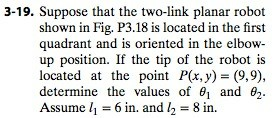# 3-19. Suppose that the two-link planar robot shown in Fig. P3.18 is located in the first...

###### Question:3-19. Suppose that the two-link planar robot shown in Fig. P3.18 is located in the first quadrant and is oriented in the elbow up position. If the tip of the robot is located at the point P(x, y)- (9,9), determine the values of θ1 and θ2. Assume 6 in. and /2 8 in

#### Similar Solved Questions

##### How do you use the quotient rule to show that 1/f(x) is decreasing given that f(x) is a positive increasing function defined for all x?
How do you use the quotient rule to show that 1/f(x) is decreasing given that f(x) is a positive increasing function defined for all x?...
##### Find the Jacobian of the transformation: x=uev, y=veu
Find the Jacobian of the transformation: x=uev, y=veu...